csharp 与 c++交互接口定义如下：

#if UNITY_IPHONE || UNITY_XBOX360
[DllImport("__Internal")]
#else
[DllImport("ptotobuf-lib")]
#endif

public static extern int iDeserial(IntPtr pb, int length);



csharp 获取 bytes 数组的首地址可以通过GCHandle来获取：

c++部分与 c#交互的部分需要写在 extern里, 参数传递指针和 bytes 数组的长度：

extern "C"
{
int iDeserial(const char* pb, int length)
{
XNet::Student student;
if (!student.ParseFromArray(pb, length))
{
printf("parse student error");
}
else
{
int age = student.age();
int num = student.num();
std::cout << "age: " << age << std::endl;
std::cout << "num:" << num << std::endl;
return age + num;
}
return 0;
}
}


1. Windows 部分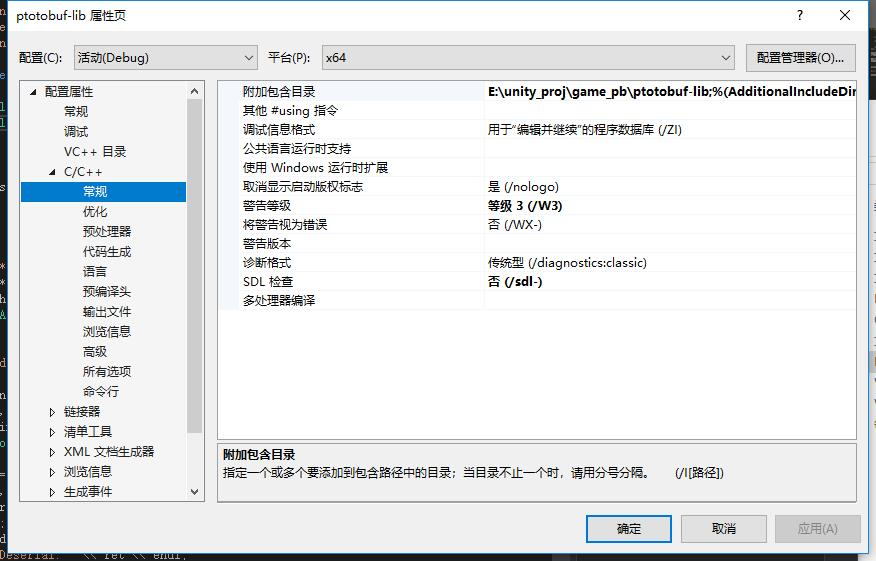1. Android 部分

APP_ABI          := armeabi-v7a x86
APP_OPTIM         := release
APP_PLATFORM      := android-14
#APP_BUILD_SCRIPT := Android.mk
APP_STL       := c++_static



LOCAL_PATH := $(call my-dir) include$(CLEAR_VARS)

LOCAL_CFLAGS:= -DHAVE_PTHREAD=1

#  c++目录的相对路径
MY_FILES_PATH  :=  $(LOCAL_PATH)/ # c++后缀 MY_FILES_SUFFIX := %.cpp %.cc %.c # 递归遍历目录下的所有的文件 rwildcard=$(wildcard $1$2) $(foreach d,$(wildcard $1*),$(call rwildcard,$d/,$2))

# 获取相应的源文件
MY_ALL_FILES := $(foreach src_path,$(MY_FILES_PATH), $(call rwildcard,$(src_path),*.*) )
MY_ALL_FILES := $(MY_ALL_FILES:$(MY_CPP_PATH)/./%=$(MY_CPP_PATH)%) MY_SRC_LIST :=$(filter $(MY_FILES_SUFFIX),$(MY_ALL_FILES))
MY_SRC_LIST  := $(MY_SRC_LIST:$(LOCAL_PATH)/%=%)

# 去除字串的重复单词
define uniq =
$(eval seen :=)$(foreach _,$1,$(if $(filter$_,${seen}),,$(eval seen += $_)))${seen}
endef

# 递归遍历获取所有目录
MY_ALL_DIRS := $(dir$(foreach src_path,$(MY_FILES_PATH),$(call rwildcard,$(src_path),*/) ) ) MY_ALL_DIRS :=$(call uniq,$(MY_ALL_DIRS)) # 赋值给NDK编译系统 LOCAL_SRC_FILES :=$(MY_SRC_LIST)
LOCAL_C_INCLUDES:= $(LOCAL_PATH)/ LOCAL_SHARED_LIBRARIES:= LOCAL_MODULE:= libprotobuf-lib LOCAL_MODULE_TAGS := optional LOCAL_LDLIBS += -llog include$(BUILD_SHARED_LIBRARY)



1. osx 部分

osx 需要把 c++代码编译成 bundle格式的库文件，需要新建一个 xcode 工程，选项如下图如下所示：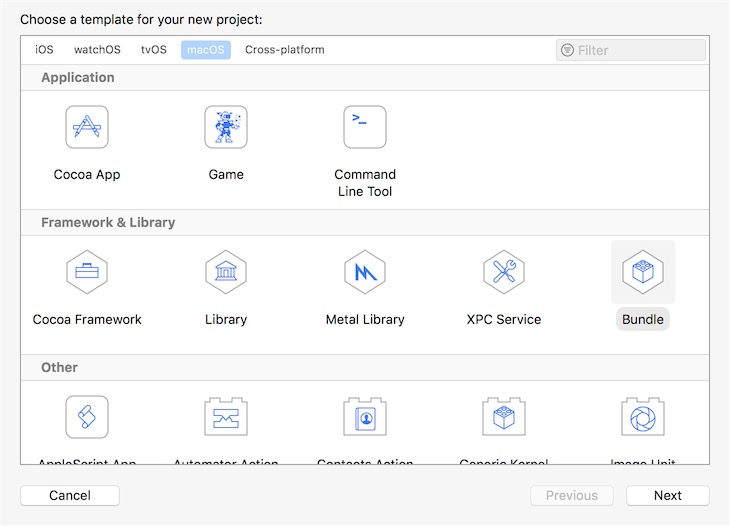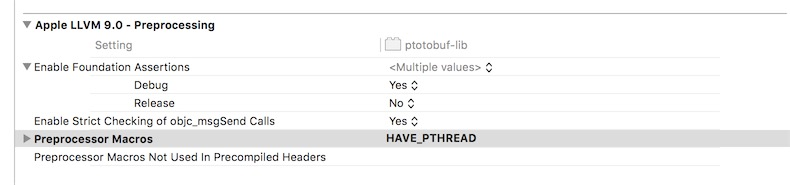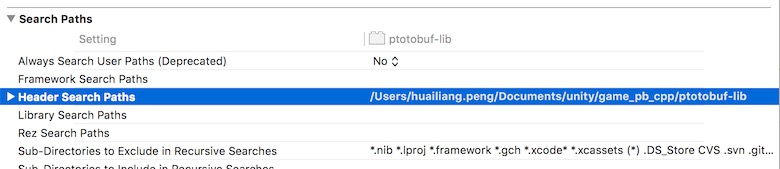1. ios 部分
osx 需要把 c++代码编译成 bundle格式的库文件，需要新建一个 xcode 工程，选项如下图如下所示：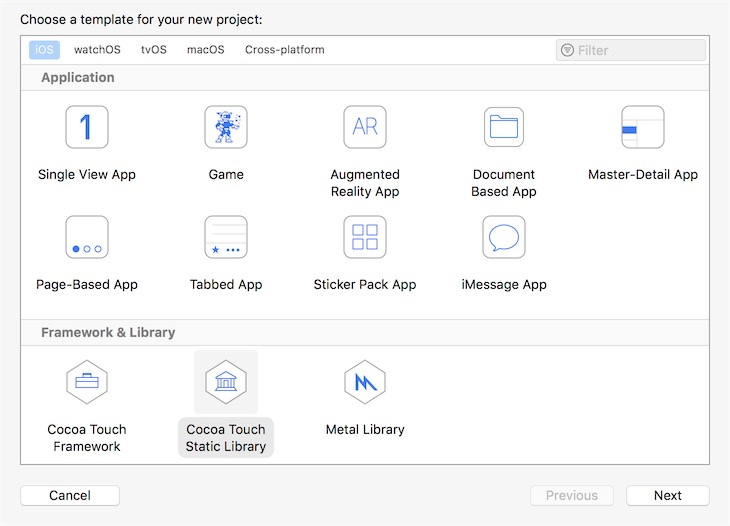ios 设置部分基本上和 osx 是一样的，记得一处地方要设置好，build 的对象要选择真机而不是模拟器，否则在真机上无法运行：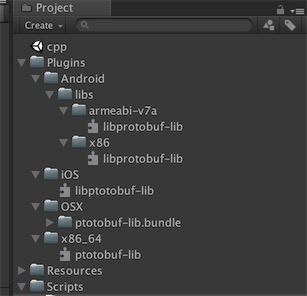**github 对应的地址，点击这里.